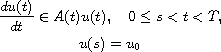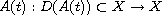Electron. J. Diff. Equ., Vol. 2011 (2011), No. 92, pp. 1-30.

### Time-dependent domains for nonlinear evolution operators and partial differential equations Chin-Yuan Lin

Abstract:in a real Banach space X, where the nonlinear, time-dependent, and multi-valued operatorhas a time-dependent domain D(A(t)). It will be shown that, under certain assumptions on A(t), the equation has a strong solution. Illustrations are given of solving quasi-linear partial differential equations of parabolic type with time-dependent boundary conditions. Those partial differential equations are studied to a large extent.Chin-Yuan Lin Department of Mathematics National Central University Chung-Li 320, Taiwan email: cylin@math.ncu.edu.tw